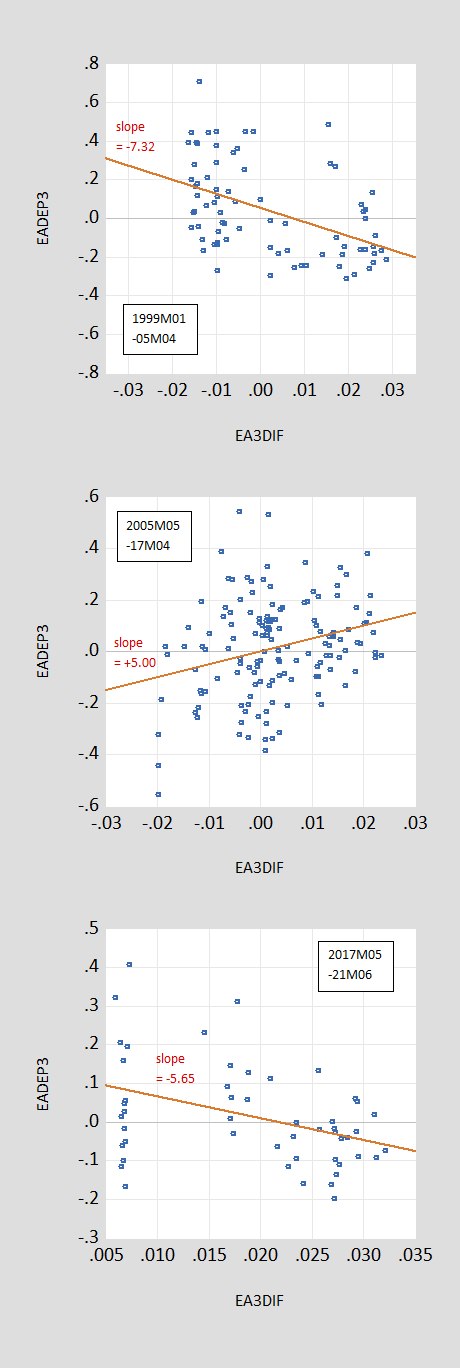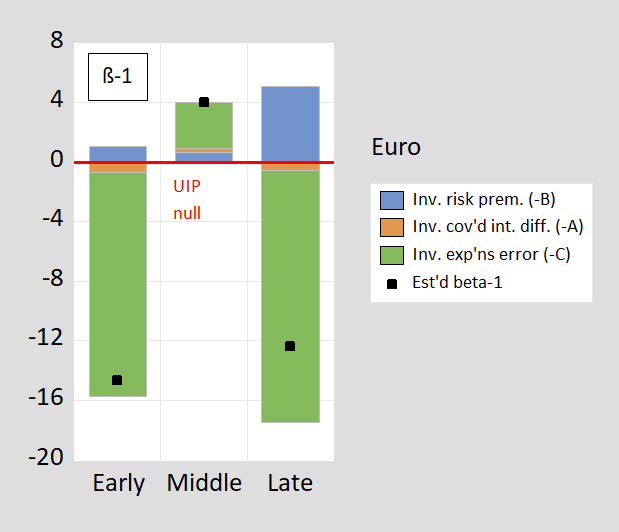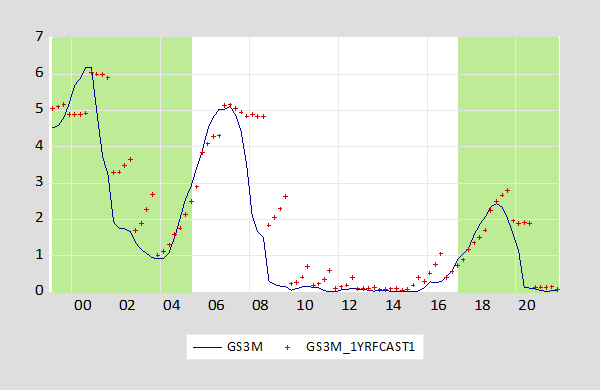### Do Exchange Rate Movements Equalize Yields?Fama (JME, 1984), and Tryon (1979) demonstrated that changes in the exchange rate  do not equal the forward premium, in what came to be known as the forward premium puzzle. Since the forward premium equals the interest differential in the absence of current and incipient capital controls and in the absence of default risk —  this finding is equivalent to the result that interest rates, after accounting for exchange rate changes, are not equalized on average.

In other words, if the yield on the US default-risk-free bond is 2% and the yield on a UK default-risk-free bond is 5%, then the US dollar does not on average appreciate by 3% against the pound in order to equalize returns. This finding could be explained, for instance, by the presence of a time-varying exchange risk premium on pound sterling assets (vs. dollar assets); however, it’s not been easy to find robust evidence of determinants of such a time varying premium.

While this puzzle has largely persisted in the ensuing 25 years, it seemingly disappeared during and after the global financial crisis — until re-appearing in recent years.Figure 1: Ex post 3 month annualized depreciation of euro/dollar (vertical axis) against 3 month offshore US-euro interest differential (horizontal axis), for 1999M01-2005M04 (top figure), for 2005M05-2017M04 (middle figure), and 2017M05-2021M06 (bottom figure). Samples pertain to interest rate data, so 1999M01-2005M04 sample includes 3 month ex post depreciation up to 2005M07.

Recall the puzzle: If the joint hypothesis of uncovered interest rate parity (UIP) and full information rational expectations –- sometimes termed the unbiasedness hypothesis — held, then the slope of the regression lines (in red) would be indistinguishable from unity. In the top panel 1999M01-2005M04), the slope coefficient is significantly different from that value at the 10% msl. (We can’t test for UIP directly because the market’s expectation of depreciation is unobserved.) This pattern of coefficient reversal holds up for other dollar-based exchange rates, as well as for other currency pairs (with a couple exceptions). The fact that the coefficient is positive in the post-global financial crisis period is what we term “the New Fama puzzle”, in a newly revised NBER Working Paper No 24342 (ungated version working paper), coauthored with Laurent Ferrara (SKEMA Business School), Matthieu Bussière (Banque de France), and Jonas Heipertz (Columbia Business School).

Uncovered interest parity is one of the most central concepts in international finance. At the same time, empirical validation of this concept has proven elusive. In fact, the failure of the joint hypothesis of uncovered interest rate parity (UIP) and rational expectations – sometimes termed the unbiasedness hypothesis – is one of the most robust empirical regularities in the literature, vigorously examined since Fama’s (1984) finding that interest rate differentials point in the wrong direction for subsequent ex-post changes in exchange rates.

The most commonplace explanations – such as the existence of an exchange risk premium, which drives a wedge between forward rates and expected future spot rates – have some empirical verification, albeit fragile (we’re investigating yields on assets like pound sterling, Japanese yen, Swiss franc, not Chinese yuan or Argentine pesos). In our explanation, we’ll take an alternative approach relating to expectations, for the reasons discussed below.

In the middle panel of Figure 1 (2005M05-2017M04) we show the slope coefficient flips to positive 5.0, significantly different from the value of unity. Finally, in the bottom panel of Figure 1 (2017M05-2021M06), the slope coefficient again flips to a negative value, of -5.65. (In the paper, we examine 1 year horizons and 1 year interest differentials, but the pattern of results are the same.)

One might be tempted to ascribe the move to a positive coefficient in the middle period to a reduction in risk; this doesn’t seem to be very plausible as the period during the financial crisis was one marked by elevated risk, certainly as measured for instance by the VIX. In any case, augmenting the regression with the VIX doesn’t change these slope coefficient estimates.

The use of survey based expectations — thereby dropping the rational expectations hypothesis — data provides the following insights. First, interest differentials and anticipated exchange rate changes are positively correlated across all the subsamples considered, consistent with the proposition that investors tend to equalize at least partially expected returns expressed in common currency terms (see also Chinn and Frankel (2020) for results 1986-2017).

Second, the switch in the β coefficient arises because the correlation of expectations errors (defined as expected minus actual) and interest differentials changes substantially between pre- and post-crisis periods. This is important, as can be seen by examining the probability limit of the β’ coefficient in a Fama regression:

s+1 – s = α’ + β'(i-i*) + error

so:

plim(β’) = 1 – [A] – [B] – [C]

Where

[A] ≡ cov(covered interest diff.,i-i*)/var(i-i*)
[B] ≡ cov(risk premium, i-i*)/var(i-i*)
[C] ≡ cov(forecast error, i-i*)/var(i-i*)

covered interest differential = – [(f – s) – (i-i*)]
risk premium = f – ε(s+1)
forecast error = ε(s+1) – s
f is the forward rate for period +1
s is the current spot exchange rate
ε(s+1) is subjective market expectations of the future spot exchange rate (proxied using Consensus Forecasts survey data).

The decomposition for the euro/dollar (β’-1) is shown in Figure 2 below, for the same subperiods (for annual horizon, as in the paper). The components are shown as theoretical β’-1 + [-A] + [-B] + [-C], so as to add up to the estimated β’-1 (which is the UIP null, red line).Figure 2: Decomposition of euro/dollar β’-1. [A] is brown, [B] is blue, [C] is green; black square denotes estimated β’, line at 0 denotes theoretical β’-1 under unbiasedness hypothesis, all for 3 month horizon. Black square denotes the OLS point estimate. Early is 1999M01-2005M04, Middle is 2005M05-2017M04 and Late is 2017M05-2021M06. Source: author’s calculations (calculations for 1 year horizon in BCFH (2022).

Exchange risk comovement with the interest differential is not the primary reason why the slope coefficient has switched to positive and then to negative (although the altered behavior of exchange risk does play a role, as shown by the changes in the blue bars). Rather, how expectations errors comove with the interest differential appears of central importance — that is the [C] component (green bar). This correlation changes because in the crisis/post-crisis period (2005M05-17M04), the dollar was weaker anticipated.

Notice that the the covered interest differential (which has widened, right after the financial crisis, and more recently as financial reforms have raised the costs of hedging) and it’s correlation with the interest differential does matter — but not very much compared to expectational errors and their comovements with interest differentials. Hence, we are not ignoring this development (remember, we are using offshore yields, so the covered interest differentials cannot be attributed to capital controls.). For more discussion, see this post, and work by Du, Tepper and Verdelhan (2018) (ungated version).

Why did this occur? We conjecture this has to do with the fact that market expectations were for a more rapid rise in US interest rates — and hence stronger dollar — than actually occurred, particularly before explicit forward guidance is implemented. This conjecture is illustrated using the actual 3 month Treasury yield (which moves very closely with the offshore 3 month yields used in the paper).Figure 3: Three month Treasury yield (blue line) and Survey of Professional Forecasters mean forecast (red +) as of Q3, in %. Green shading denotes early, late periods. Source: Federal Reserve Board, Philadelphia Fed.

Finally, notice in our late period, after liftoff in US rates from the zero lower bound, as interest rate expectations match more closely what occurs, the original negative coefficient re-appears. This finding is an addition to our findings previously described in our earlier (2019) post.

One major implication of our findings — which transcends the findings of what the regression coefficient is now versus then — is that the characteristics of exchange rate expectations matter a lot, possibly more than risk attributes of highly substitutable (for dollar) assets.

The ungated version of the paper, here.